# Chow Pressure Group (CPG)

## 1. Theory

Chu et al (2017) showed that there is a relationship between the magnitude of pressure interference (MPI) and the stabilized Chow Presure Group (CPG) value

The CPG calculation considers the bottomhole pressure trend before and after a well is put on production (POP). Fig. 1 shows an example of this, in which the dashed line represents the pre-POP bottomhole pressure trend. The pressure difference, $$\Delta p$$, is the difference between the forecasted "prior" bottomhole pressure trend and the measured bottomhole pressure (that starts when the offset well is put on production).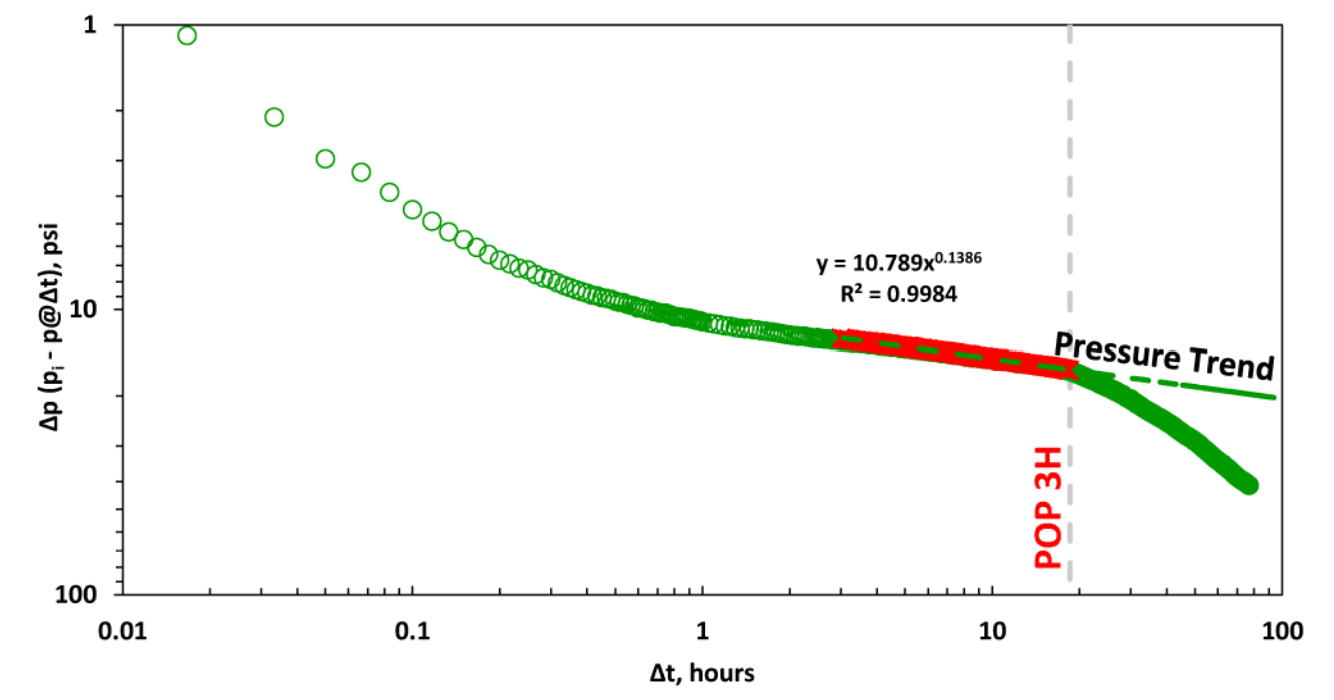Fig. 1: Example of interference impact when offset well is put on production (POP), Chu et al (2018).

$$\Delta p'$$ may be one of the following -

### 1.1 Derivative Options

The logarithmic derivative used in well testing applied directly on the $$\Delta p$$ data is given by, i.e., .

There are three different ways to compute this derivative in whitson+:

• The Bourdet Derivative (Bourdet et al., 1989)
• Weighted Central Difference
• Central Difference

The Bourdet derivative is commonly used in well-test analysis (PTA) for identifying flow regimes (Lee et al., 2003) and has similarly found utility in RTA.

#### 1.1.1 Governing Equation

To calculate the Bourdet derivative (Bourdet et al., 1989) at any given point, one point before (left) and one point after (right) is used.

#### 1.1.2 Smoothing

The Bourdet approach is illustrated in Fig. 2 and is a way to calculate and smooth the derivative based on a log-cycle window size, L.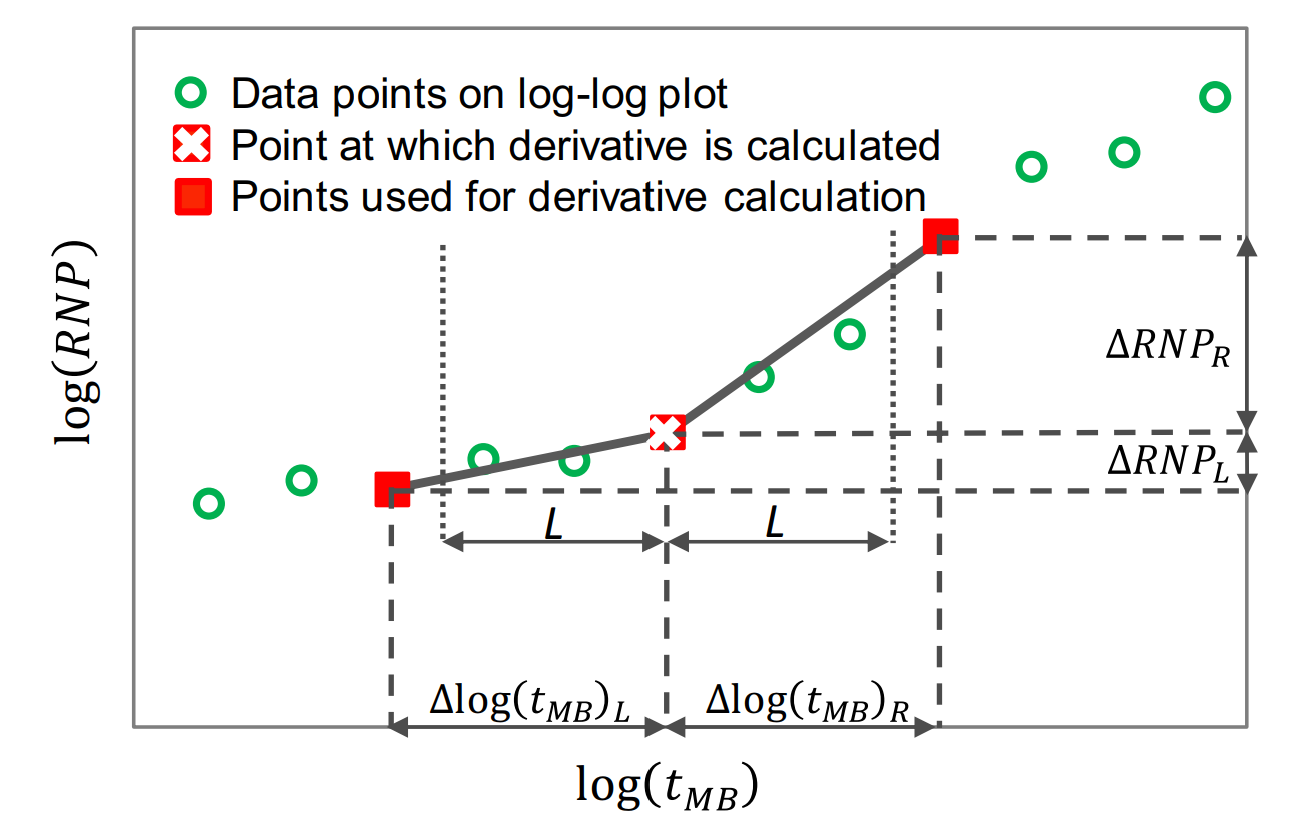Fig. 2: Illustration of a derivative calculating using the Bourdet method. Image courtesy of Samaneh Moghadam (April, 2020). Modified from Lee et al. (2003).

It is different from a weighted central difference in the way that it uses a constant log-cycle window size, L, on both sides of the point of derivation. A weighted central difference, on the other hand, uses a constant index step size, e.g. i-5 and i+5 for step size of 5, on each side of the point of derivation. The window size, L, represents the log-cycle fraction used to control the amount of smoothing. Typical values are 0.01 to 0.2. The default L in whitson+ is 0.2. The larger the L value, the more smoothing.

The Bourdet algorithm implemented in whitson+ was provided by Behnam Zanganeh.

### 1.2 Weighted Central Difference

The weighted central difference is given by,

where the $$L$$-subscript refers to the index to the left with a constant step size, $$S$$, i.e. $$i-S$$ and the $$R$$-subscript refers to the index to the right with a constant step size, $$S$$, i.e. $$i+S$$.

### 1.3 Central Difference

This is the naive implementation, given by three points per derivative for central difference in the following manner

### 1.4 Leveraging the Pressure Integral

An alternative approach is to use the pressure integral as the basis to calculate the $$\Delta p$$. Here, the $$\Delta p$$ is rewritten as an integral as $$\Delta p_{integral}$$ - which is implemented in whitson+ as Then, the typical well testing derivative is applied to the $$\Delta p_{integral}$$ data as, The integral method preserves the signature of the pressure plot with respect to time while significantly reducing the the noise in the derivatives. This method allows for better stabilization of the derivative values despite noisy pressure data and hence is used for more interpretability in CPG plots.

## 2. Level of Interference

CPG value Level of Interference
0 No pressure interference
0-0.5 Weak
0.5-0.8 Moderate
0.8-1 Strong

## 3. Data Requirements and Averaging

To perform this calculation, you only need date (time) and bottomhole pressure data. We recommend averaging the data on a 15 minute basis to remove noise in the data.

## 4. Example: Drawdown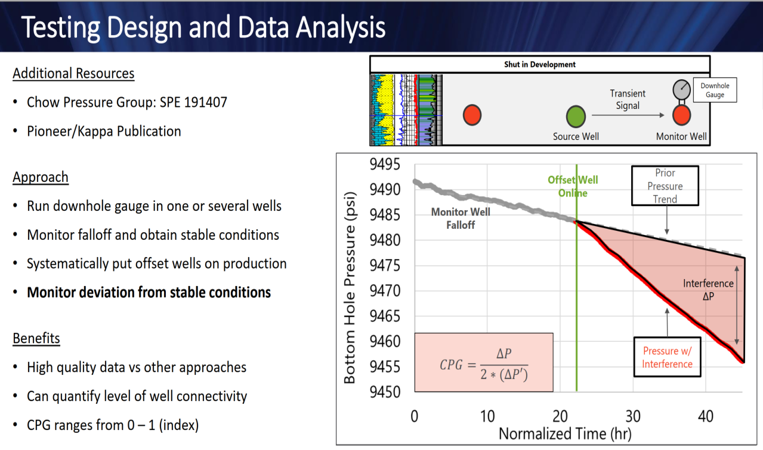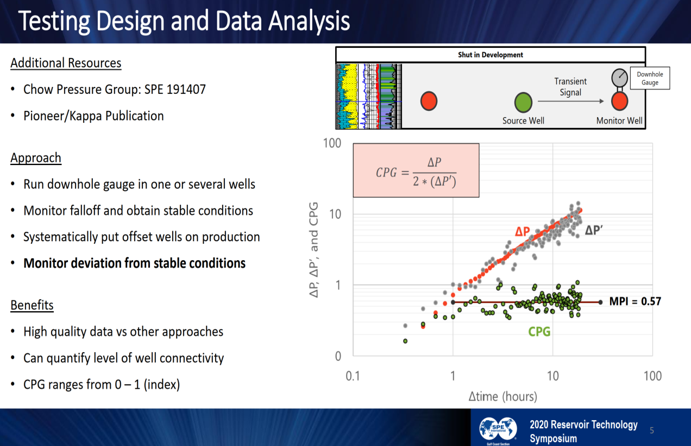Fig. 3: Drawdown Example (Hart & Ingle, 2020)

## 5. Example: Buildup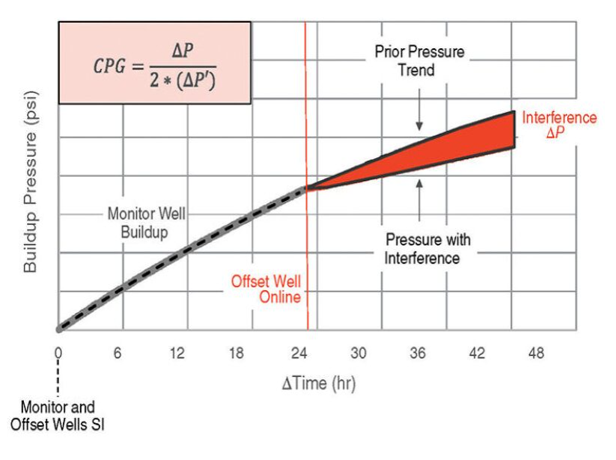Fig. 4: Drawdown Example (JPT, 2020)

Note

The Chow Pressure Group is not only a convenient way to check the slopes of log-log straight lines, but also means a identify various flow regimes. For example, the slope of 1/2 on the log-log plot may indicate a linear flow regime, which would result in a CPG of one. Likewise, the bilinear flow regime has a slope of 1/4, which would result in a CPG value of 2.

## 6. CPG in whitson+

Here is an example dataset to try it for yourself! Download the CPG Example Dataset here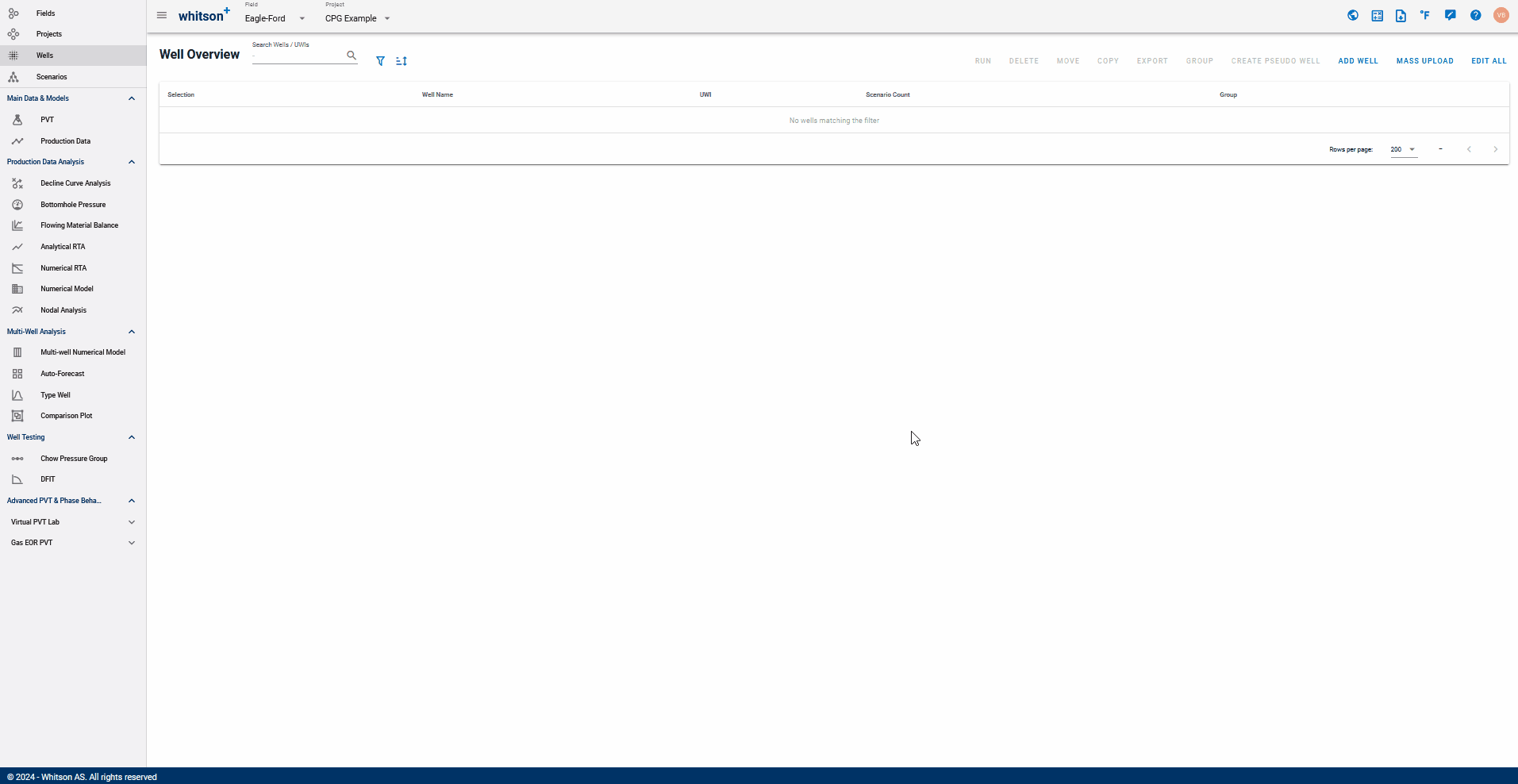You can analyze the Chow Pressure Group for the monitoring well in whiston+ by navigating to the Chow Pressure Group feature under Well Testing.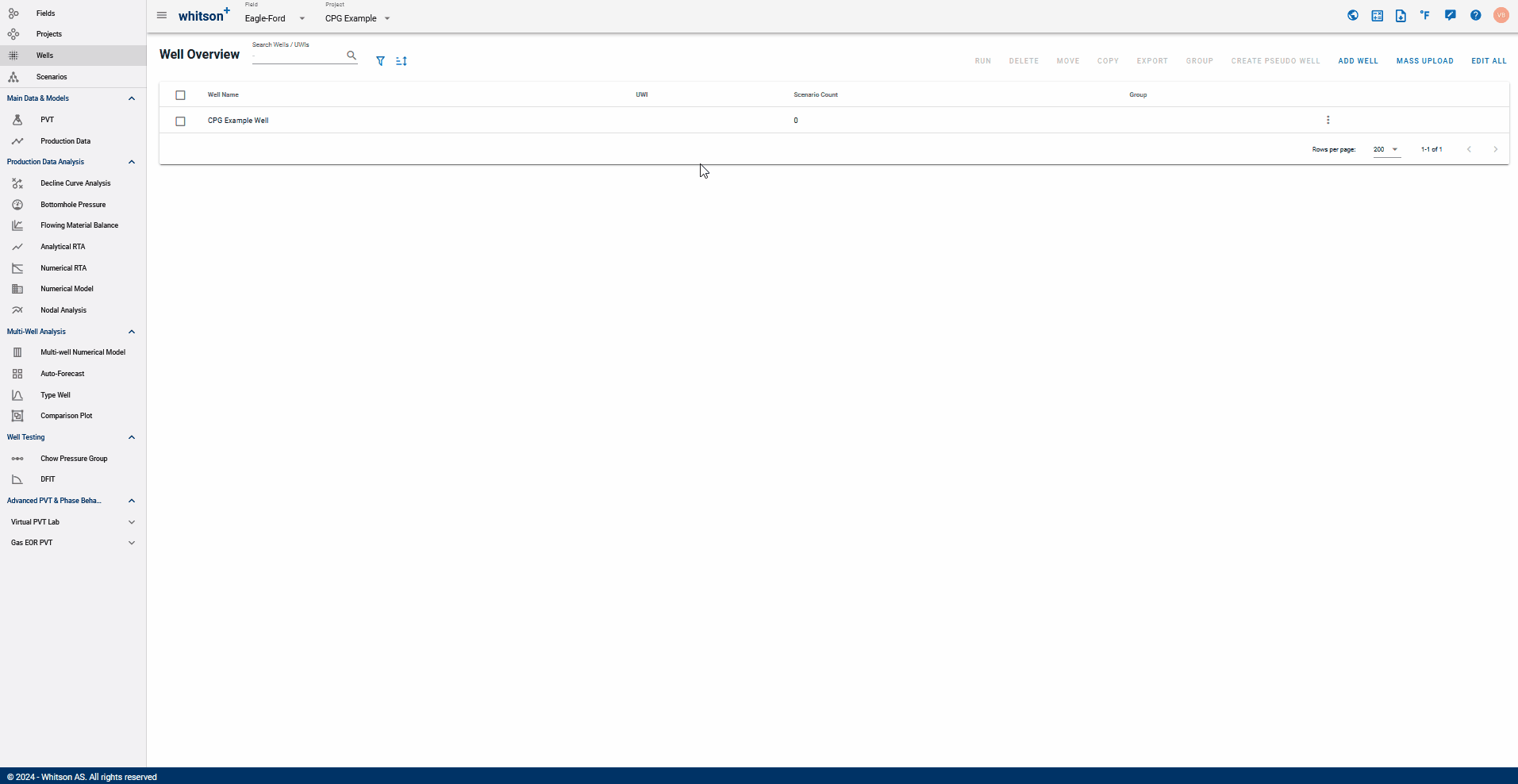On the Pressure Difference plot on the top-left,

1. Move the CPG fit line (yellow, dashed line) to match the pressure data prior to the production well POP date. You can also adjust the slope by manually changing the Constant and Exponent.
2. Adjust the dashed vertical line along the monitoring well data to the day when production well is POP.
3. The difference in these CPG fit and the data is plotted along with it's derivative as $$\Delta p, \Delta p'$$ vs. time (bottom-right). Observe the changes in this plot as the Fit line is changed.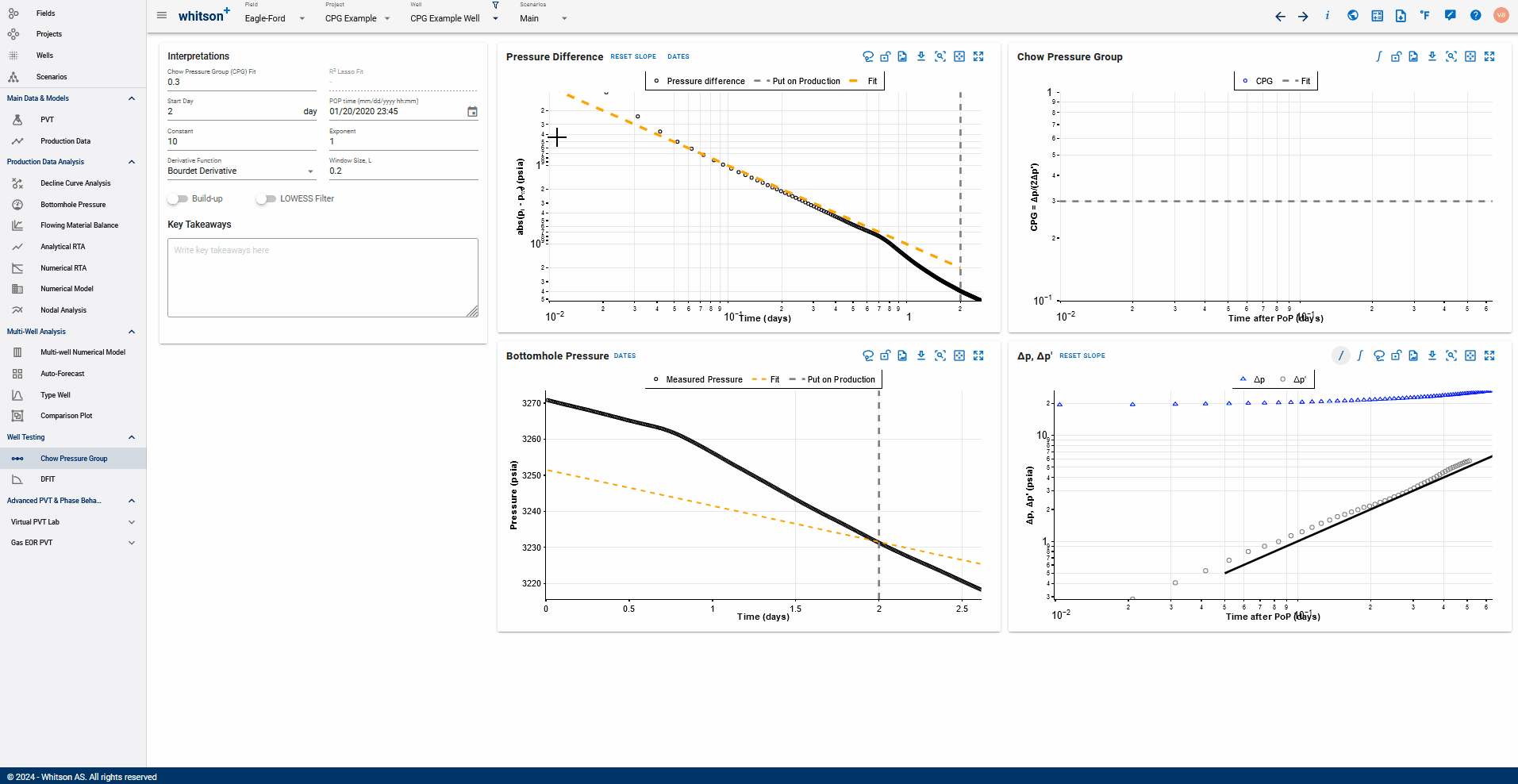On the $$\Delta p, \Delta p'$$ vs. time plot (bottom-right),

You can switch between the derivative calculation techniques mentioned above - by selecting from the dropdown list for 'Derivative Function'.

Bourdet Derivative and Weighted Central Difference give you the additional option to modify the smoothing window as a log cycle fraction or a step size respectively.

Both these methods are higher in accuracy compared to simply using the Central Difference with a fixed step size.

Notice how switching between the different methods and window sizes in the GIF below changes the derivative shape.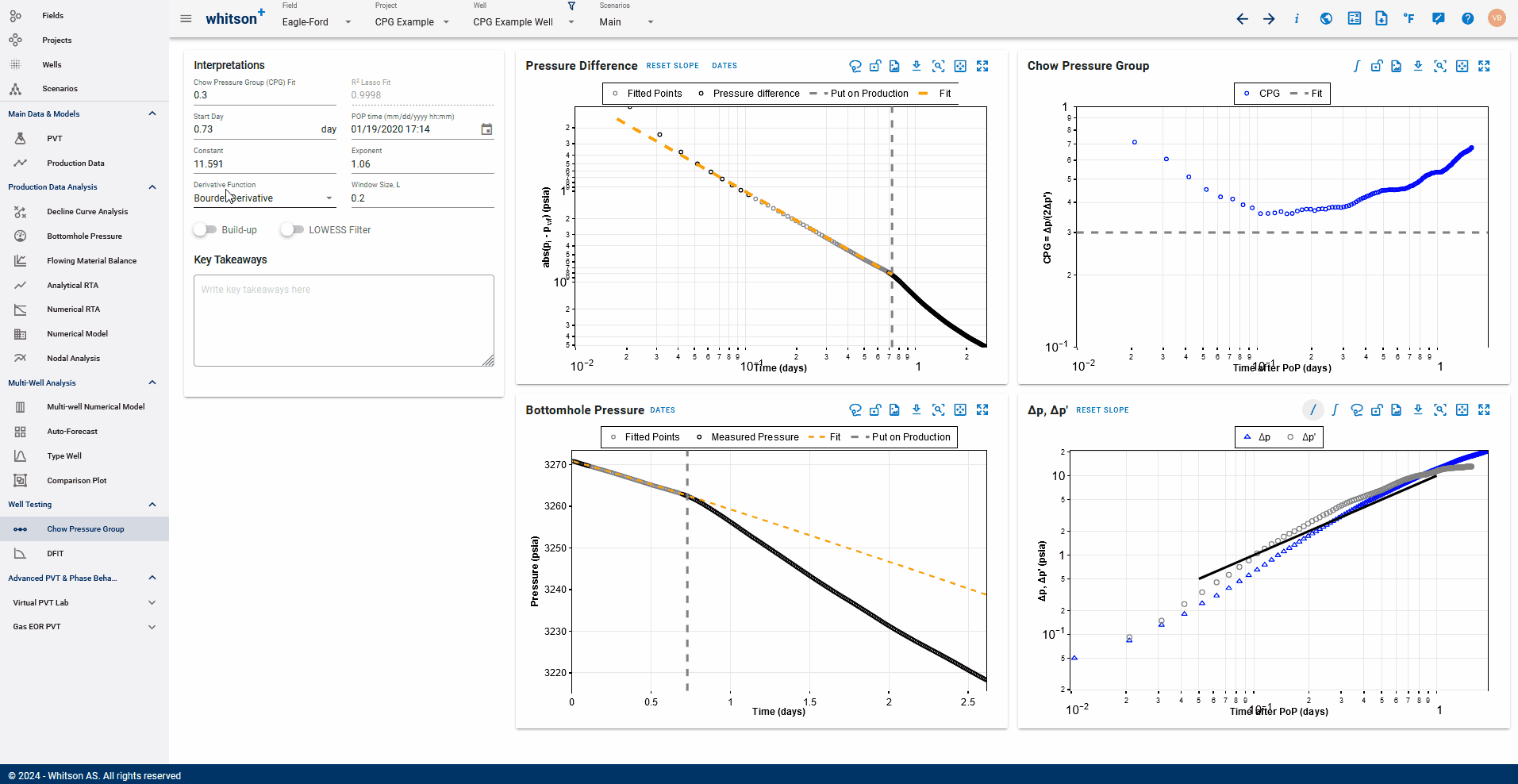Toggle the switch 'Use Pressure Integral' in the plot to recalculate the pressure as an integral and recompute the derivative based on the derivative function selected above.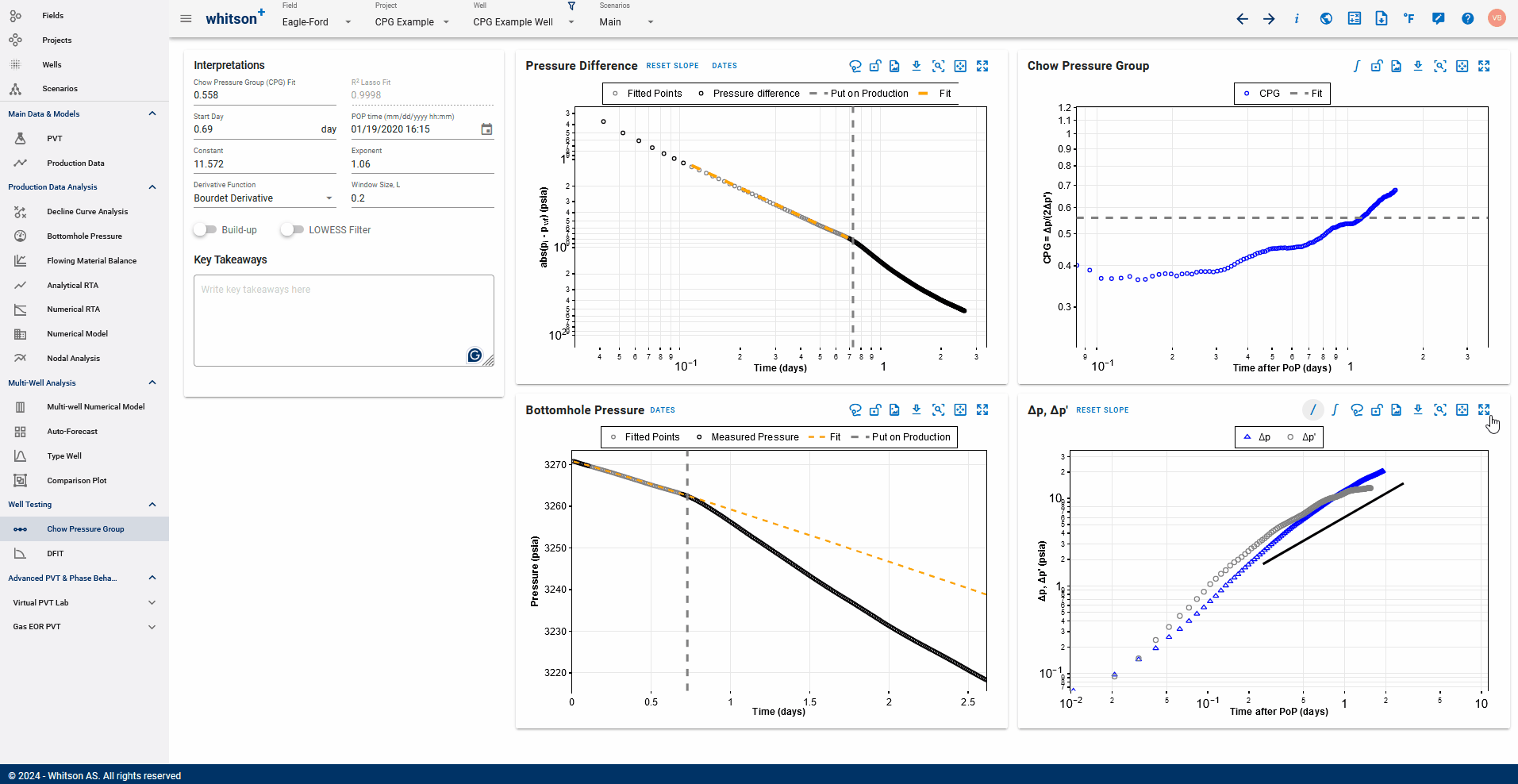This method inherently smooths the pressure response, and removes additional noise that may be a part of the dataset to create a much smoother derivative and CPG plot. Since the pressure integral is already smooth, a smaller window size can be used for derivative calculation.

Next, on the Chow Pressure Group plot, move the horizontal dashed line to track the CPG values after POP date. You can also adjust it by entering a value for CPG fit.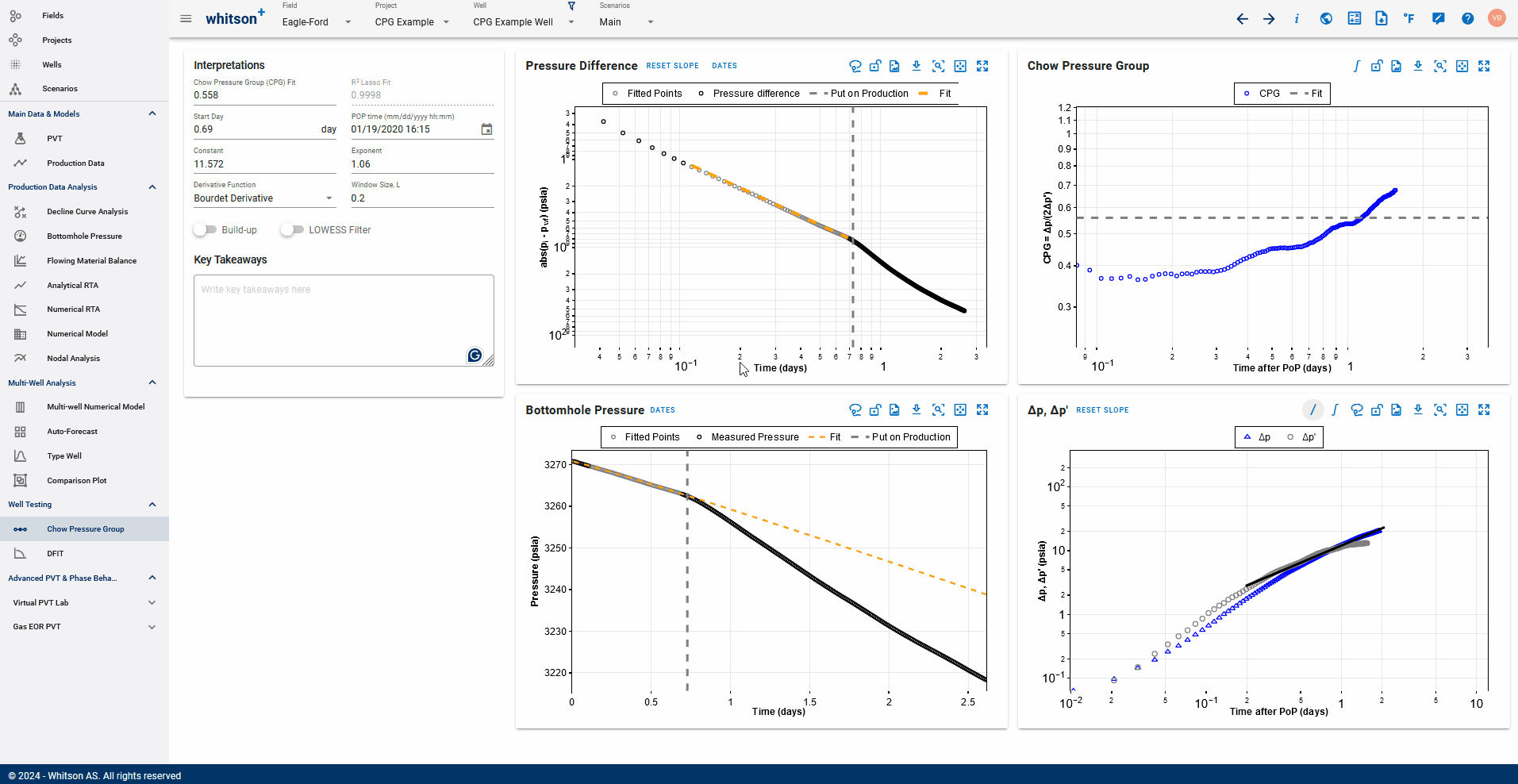This is the CPG value that indicates the level of interference between the production well and the monitoring well.

 [Christopher R. Clarkson. Unconventional Reservoir Rate-Transient Analaysis. Volume One. Fundamentals, Analysis Methods and Workflow.]

 [Lee, J., Rollins, J.B., Spivey, J.P., 2003. Pressure Transient Testing. SPE, Richardson, TX.]